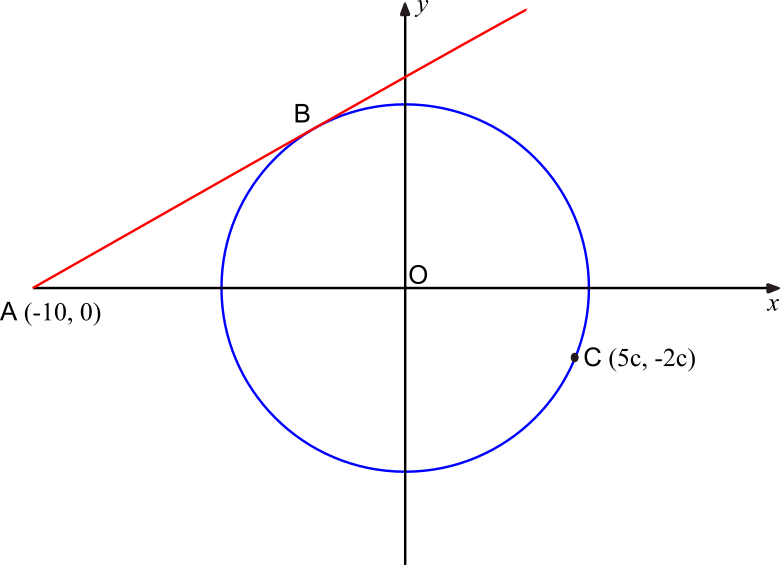# Exam-Style Questions on Geometry

## Problems on Geometry adapted from questions set in previous Mathematics exams.

### 1.

GCSE Higher

The diagram shows a circle with its centre at the origin.The red line AB is a tangent to the circle at the point B.

Angle OAB is 30°.

Point A has coordinates $$(-10, 0)$$

Point C has coordinates $$(5c, -2c)$$

Find the value of $$c$$ giving your answer correct to three significant figures and showing all of your working.

### 2.

IB Studies

The vertices of quadrilateral ABCD are A (2, 4), B (-1, 5), C (–3, 4) and D (–2, 2).

(a) Calculate the gradient of line CD.

(b) Show that line AD is perpendicular to line CD.

(c) Find the equation of line CD. Give your answer in the form $$ax+by=c$$ where $$a,b,c\in \mathbf Z$$

Lines AB and CD intersect at point E.

(d) Find the coordinates of E.

(e) Find the distance between A and D.

The distance between D and E is $$\sqrt{20}$$.

(f) Find the area of triangle ADE.

If you would like space on the right of the question to write out the solution try this Thinning Feature. It will collapse the text into the left half of your screen but large diagrams will remain unchanged.

The exam-style questions appearing on this site are based on those set in previous examinations (or sample assessment papers for future examinations) by the major examination boards. The wording, diagrams and figures used in these questions have been changed from the originals so that students can have fresh, relevant problem solving practice even if they have previously worked through the related exam paper.

The solutions to the questions on this website are only available to those who have a Transum Subscription.

Exam-Style Questions Main Page

Search for exam-style questions containing a particular word or phrase:

To search the entire Transum website use the search box in the grey area below.